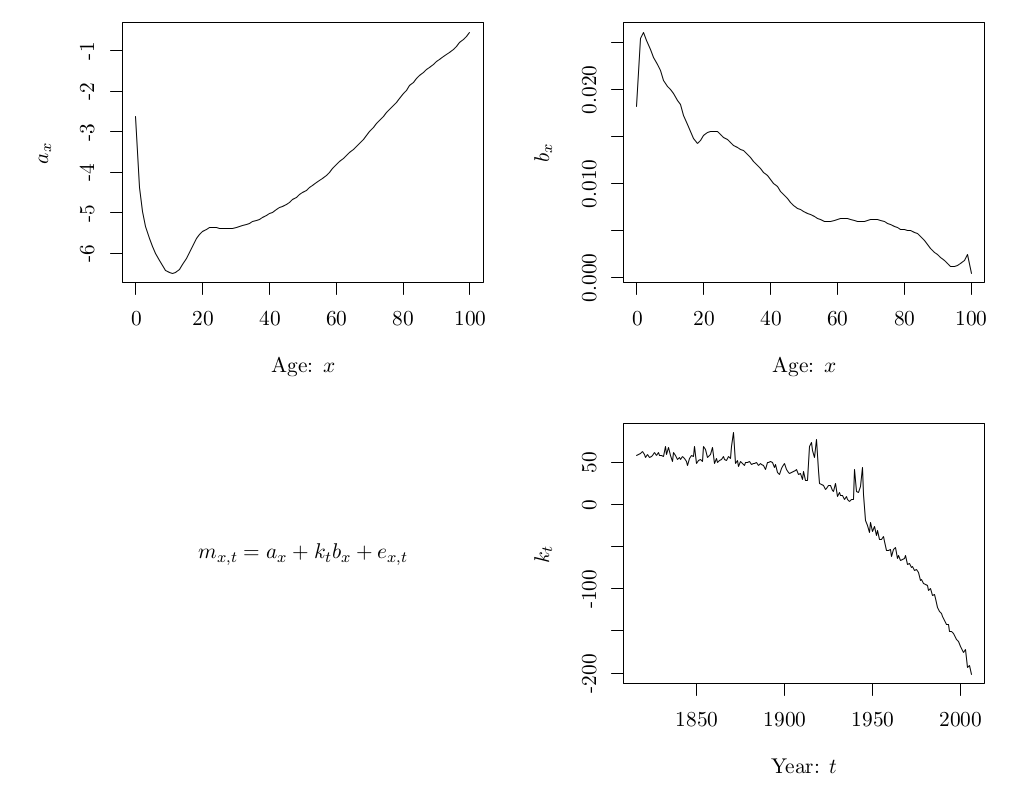Want to share your content on R-bloggers? click here if you have a blog, or here if you don't.

I’ve always struggled with using `plotmath` via the `expression` function in R for adding mathematical notation to axes or legends. For some reason, the most obvious way to write something never seems to work for me and I end up using trial and error in a loop with far too many iterations.

So I am very happy to see the new latex2exp package available which translates LaTeX expressions into a form suitable for R graphs. This is going to save me time and frustration!

Here is a quick example showing a Lee-Carter decomposition of some mortality data.

``````library(demography)
library(latex2exp)

fit <- lca(fr.mort)

par(mfrow=c(2,2), mar=c(4,5,2,1), family="serif")
plot(fit\$age, fit\$ax, type="l", ylab=TeX("a_x"), xlab="Age: x")
plot(fit\$age, fit\$bx, type="l", ylab=TeX("b_x"), xlab="Age: x")
plot(0, type="n", axes=FALSE, xlab="", ylab="")
text(1, 0, TeX("m_{x,t} = a_x + k_tb_x + e_{x,t}"))
plot(fit\$kt, ylab=TeX("k_t"), xlab="Year: t")``````There are several more examples in the package documentation.

The results are still a little ugly, but that is because of the limitations of base graphics in R. To get something more LaTeX-like, the tikzDevice package can be used as follows.

``````library(demography)
library(tikzDevice)

fit <- lca(fr.mort)

tikz("tikz-test.tex",width=15/2.54,height=12/2.54)
par(mfrow=c(2,2),mar=c(4,5,2,1),family="serif")
plot(fit[["age"]],fit\$ax,type="l",
ylab="\$a_x\$", xlab="Age: \$x\$")
plot(fit[["age"]],fit\$bx,type="l",
ylab="\$b_x\$", xlab="Age: \$x\$")
plot(0,type="n",axes=FALSE,xlab="",ylab="")
text(1,0,"\$m_{x,t} = a_x + k_tb_x + e_{x,t}\$")
plot(fit\$kt,ylab="\$k_t\$", xlab="Year: \$t\$")
dev.off()``````While the results look much nicer, it is rather slow.